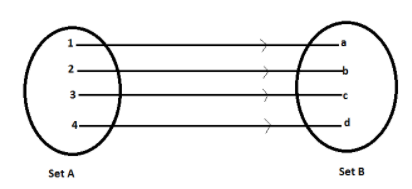QuestionAnswers

# One mapping is selected at random from all the mappings of the set A=1, 2, 3, ....,n into itself. The probability that the mapping selected is one to one, is given by a. $\dfrac{1}{{{n^n}}}$ b. $\dfrac{1}{{n!}}$c. $\dfrac{{(n - 1)!}}{{{n^{n - 1}}}}$d. None of these.Hint: Here in this question you need to know two concepts one is probability and other is one to one mapping.
Probability: -
Probability=Favourable outcomes/Total number of outcomes.
Probability can never be greater than one.
One to one mapping: -
One to one or (1-1) function is a relation that preserves the “uniqueness”. Every unique member of the function’s domain is mapped to the unique member of the function’s range. This mapping is sometimes also called as injective mapping.

Complete step-by-step answer: Here given set is A=1, 2, 3, ...., n
Number of ways first element of set A can be mapped = n
Number of ways second element of set A can be mapped = n
Number of ways the third element of set A can be mapped = n and so on.
Total number of mapping from set A to itself=$n \times n \times ...... \times n = {n^n}$ (it is being multiplied to n times)
For one to one mapping: -
Number of ways to map first element in set A=n
Number of ways to map second element in set A=n-1
Number of ways to map third element in set A=n-2
Number of ways to map nth element in set A=1
Total number of one to one mapping from set A to itself=$n \times (n - 1) \times (n - 2)..... \times 1 = n!$
$\therefore$ Required probability=Total number of one to one mapping from set A to itself/Total number of mapping from set A to itself
$\Rightarrow \dfrac{{n!}}{{{n^n}}} = \dfrac{{n(n - 1)!}}{{{n^n}}}$ (Opening factorial using formula$n! = n(n - 1)(n - 2)(n - 3) \times ....... \times 3 \times 2 \times 1$ )
$\therefore \dfrac{{(n - 1)!}}{{{n^{n - 1}}}}$ (Using identity $\dfrac{{{x^a}}}{{{x^b}}} = {x^{a - b}}$ )
Hence option C is correct.

Note: Most of the times students got confused in one to one mapping so here is some easy way to understand it using this diagram.View Notes
The Difference Between an Animal that is A Regulator and One that is A ConformerCBSE Class 12 Maths Chapter-13 Probability FormulaOne to One FunctionWhat is the Cell Envelope?What is the Placebo EffectCBSE Class 12 Maths FormulasCBSE Class 8 Maths Chapter 2 - Linear Equations in One Variable FormulasWhat is the full form of phd?What is the Difference Between Trade and Commerce?CBSE Class 12 Maths Chapter-12 Linear Programming FormulaImportant Questions for CBSE Class 12 Maths Chapter 13 - ProbabilityImportant Questions with Answers for CBSE Class 6 to 12 - All SubjectsCBSE Class 6 Science Food: Where Does It Come From WorksheetsImportant Questions for CBSE Class 8 Maths Chapter 2 - Linear Equations in One VariableImportant Questions for CBSE Class 11 Accountancy Chapter 11 - Accounts From Incomplete RecordsImportant Questions for CBSE Class 12 Maths Chapter 12 - Linear ProgrammingImportant Questions for CBSE Class 12 Chemistry Chapter 1 - The Solid StateImportant Questions for CBSE Class 11 English Snapshots Chapter 4 - Albert Einstein at SchoolImportant Questions for CBSE Class 6 Science Chapter 1 - Food: Where Does It Come FromNCERT Books for Class 6 to 12 - All SubjectsCBSE Class 12 Maths Question Paper 2020Maths Question Paper for CBSE Class 12 - 2013Previous Year Question Paper for CBSE Class 12 Maths - 2014CBSE Previous Year Question Papers Class 12 Maths with SolutionsMaths Question Paper for CBSE Class 12 - 2016 Set 1 CMaths Question Paper for CBSE Class 12 - 2016 Set 1 EMaths Question Paper for CBSE Class 12 - 2016 Set 1 SCBSE Class 12 Maths Question Paper 2018 with Solutions - Free PDFCBSE Class 12 Maths Question Paper 2015 with Solutions - Free PDFMaths Question Paper for CBSE Class 12 - 2016 Set 1 NNCERT Solutions for Class 12 Maths ExercisesNCERT Solutions for Class 12 English Kaliedoscope Poetry Chapter 6 The Wild Swans at CooleRD Sharma Class 12 Maths Solutions Chapter 32 - Mean and Variance of a Random VariableCBSE Class 8 Maths Chapter 2 Linear Equation in One Variable Exercise 2.5NCERT Solutions for Class 6 Maths ExercisesNCERT Solutions for Class 10 Maths ExercisesNCERT Solutions for Class 8 Maths ExercisesNCERT Solutions for Class 7 Maths ExercisesNCERT Solutions for Class 11 Maths ExercisesNCERT Solutions for Class 9 Maths Exercises